A small spherical solid ball is dropped in a viscous liquid. Its journey in the liquid is best described in the figure by :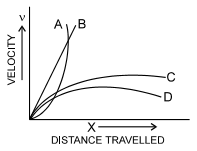1.  Curve A

2.  Curve B

3.  Curve C

4.  Curve D

Concept Questions :-

Stokes' law
High Yielding Test Series + Question Bank - NEET 2020

Difficulty Level:

Water flows through a frictionless duct with a cross-section varying as shown in the figure. Pressure p at points along the axis is represented by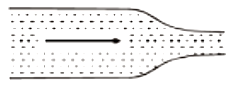1.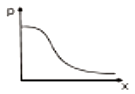2.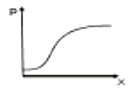3.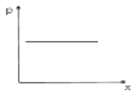4.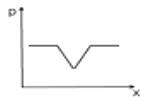Concept Questions :-

Equation of continuity
High Yielding Test Series + Question Bank - NEET 2020

Difficulty Level:

The area of cross-section of the wider tube shown in the figure is  If a mass of 12 kg is placed on the massless piston, the difference is heights h in the level of water in the two tubes is :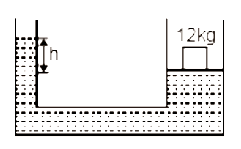1.  10 cm

2.  6 cm

3.  15 cm

4.  2 cm

Concept Questions :-

Properties of fluids
High Yielding Test Series + Question Bank - NEET 2020

Difficulty Level:

In the figure below is shown the flow of liquid through a horizontal pipe. Three tubes A, B, and C are connected to the pipe. The radius of the pipe at A, B, and C is respectively 2 cm, 1 cm, and 2 cm. It can be said that the :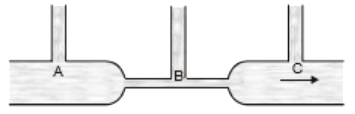1.  Height of the liquid in the tube A is maximum

2.  Height of the liquid in the tubes A and B is the same

3.  Height of the liquid in all the three tubes is the same

4.  Height of the liquid in the tubes A and C is the same

Concept Questions :-

Bernoulli theorem
High Yielding Test Series + Question Bank - NEET 2020

Difficulty Level:

Equation of continuity based on :

1.  Conservation of mass

2.  Conservation of energy

3.  Conservation of angular momentum

4.  None of these

Concept Questions :-

Equation of continuity
High Yielding Test Series + Question Bank - NEET 2020

Difficulty Level:

Bernoulli's theorem is based on :

1.  conservation of energy

2.  conservation of mass

3.  conservation of momentum

4.  conservation of angular momentum

Concept Questions :-

Bernoulli theorem
High Yielding Test Series + Question Bank - NEET 2020

Difficulty Level:

Two spherical soap bubbles of radii r1 and r2 in vaccum collapse under isothermal condition. The resulting bubble has radius equal to :

1.

2.

3.

4.

Concept Questions :-

Surface tension
High Yielding Test Series + Question Bank - NEET 2020

Difficulty Level:

The energy needed in breaking of a drop of liquid of radius R into n drops of radius r is given by (T is surface tension and P is atmospheric pressure) :

1.  $\left(4{\mathrm{\pi r}}^{2}\mathrm{n}-4{\mathrm{\pi R}}^{2}\right)\mathrm{T}$

2.  $\left(4/3{\mathrm{\pi r}}^{2}\mathrm{n}-4/3{\mathrm{\pi R}}^{3}\right)\mathrm{T}$

3.  $\left(4{\mathrm{\pi R}}^{2}\mathrm{n}-4{\mathrm{\pi r}}^{2}\right)\mathrm{T}$

4.  $\left(8{\mathrm{\pi r}}^{2}\mathrm{n}-8{\mathrm{\pi R}}^{2}\right)\mathrm{T}$

Concept Questions :-

Surface tension
High Yielding Test Series + Question Bank - NEET 2020

Difficulty Level:

A tank is filled with water up to a height H. A hole is made at a depth h below the free surface of the water in the tank. The water coming out of the orifice strikes at a distance S from the walls of the tank. Then S is given by :

Concept Questions :-

Equation of continuity
High Yielding Test Series + Question Bank - NEET 2020

Difficulty Level:

An incompressible liquid travels as shown in the figure. The speed of the fluid in the lower branch will :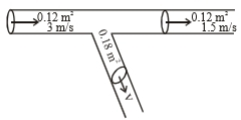1. 1 m/s

2. 1.5 m/s

3. 2.25 m/s

4. 3 m/s

Concept Questions :-

Equation of continuity